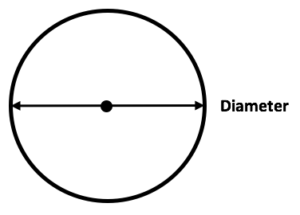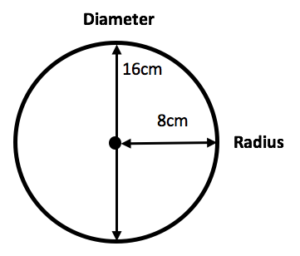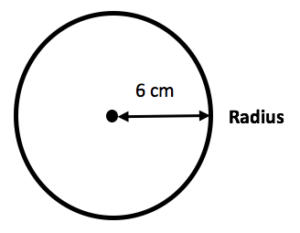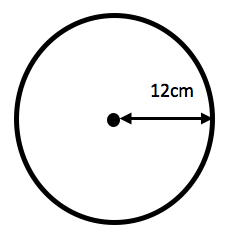What is the diameter? The diameter is the length of the line through the centre of a circle.

The diameter is always twice the length of the radius of a circle.## When do children learn about the diameter?

After learning about 2D and 3D shapes in Years 1 and 2, children will be introduced to the different parts of a circle in Year 6.

Teachers will ask children to measure the radius and diameter of different circles and may give them string so that they can measure the circumference of a circle.

Children may also be given practice questions where they are given the radius of the circle and asked to find the diameter. This will help them learn that the diameter is the radius x 2.Here is an example practice question:

What is the diameter of the circle below?Children should be able to work out the answer as they know that the diameter is the radius x 2 (r²).

6 x 2 = 12cm

Diameter = 12cm.

## Why do children need to know about the diameter?

Learning the different parts of a circle is important so that children can go on to calculate the area and circumference of a circle.

Understanding the relationship between the diameter and the radius is important when children are given practice questions.

Teachers may introduce children to the formula to calculate the circumference (C= 2 x π x r) and the formula to calculate the area (A=π x r ²). Here is a practice question calculating the circumference:

Sam’s plate has a radius of 12cm. What is the circumference of the plate?First, it is helpful to draw out a rough circle and annotate it with any measurements the question provides.

We are told that the radius of the plate is 12cm, so we can now substitute this into the formula C  =  2 x π x r (where π = 3.14).

2 x 3.14 x 12 = 75.36 cm. The circumference of Sam’s plate is 75.36 cm.

Children could have also got the same answer with the formula C = π x d, by using their knowledge that the diameter is twice the size of the radius (2 x 12 = 24).

3.14 x 24 = 75. 36 cm.

## How does Learning Street help children with the diameter?

This is one of the many occasions where our specialised maths courses will develop a previous idea, in this case, the radius, and then progress the child’s learning, in this case onto the diameter. The child will be taught about the diameter, given practice on it in order to ensure a solid understanding and also have revision on it later so that it isn’t forgotten.

Tests could include SATs, competitive 11 Plus tests or selective Independent school exams.

## Our Courses

Click through to review the courses we have available

##### Get Started

Learning Street structured courses make home study easier and more successful. There's no need to wonder which books to buy or what to do next. Everything is planned for you.

• Proven to deliver excellent results
• Used by families, private tutors and schools
• Fully planned and structured